1800-1023-196

+91-120-4616500

# Mole Fraction

## Mole Fraction (X)

Mole fraction may be defined as the ratio of number of moles of one component to the total number of moles of all the components (solvent and solute) present in the solution. It is denoted by the letter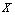. It may be noted that the mole fraction is independent of the temperature. Mole fraction is dimensionless. Let us suppose that a solution contains the components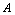and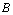and suppose that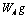ofand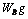ofare present in it.

Number of moles ofis given by,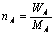and the number of moles ofis given by,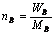where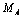and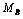are molecular masses ofandrespectively.

Total number of moles ofand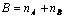Mole fraction of,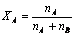Mole fraction of,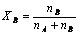The sum of mole fractions of all the components in the solution is always one.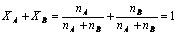.

Thus, if we know the mole fraction of one component of a binary solution, the mole fraction of the other can be calculated.

## NEET & AIIMS Exam Sample Papers

 AIIMS SAMPLE PAPERS View More NEET SAMPLE PAPERS View More